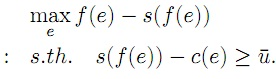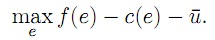#### Contracts and Moral Hazard

Contracts and Moral Hazard:

An employer who wants an employee to work hard for him should structure the compensation (wage or salary) in order to give him incentives to do just that. To hire someone and to pay him a lump-sum fee works if the task is well defined and limited, and result can be easily verified. But if the result depends on effort (e) exerted by the employee, it usually is a very bad arrangement. Let output q be a function of effort:

q = f(e),

Let’s set the price of output equal to 1, so q is also the value of output. The salary you (the principal) pays to the employee (the agent), is s(q), i.e., it depends on output, and therefore on the agent’s effort. Your problem is to choose s(q) to maximize your net profit: Πnet = q − s(q). However, you can dictate an effort level (or output level), but it is the agent that chooses his/her effort. Effort is costly to the agent; we assume that it is an increasing function c(e). The total surplus is divided between the principal and the agent: Πtot =  Πp+ Πa = f(e)−s(q)+s(q)−c(e). Note that the salary the principal pays the agent is just a transfer and it cancels out between the two. For productive efficiency total profit, or surplus, should be maximized, which in this case is:

Πtot = q − c(e),
= f(e) − c(e).

The solution is simple; it is that the agent should put in effort to the point there the marginal benefit, f′(e), is equal to marginal cost, c′(e).

You generally cannot pay the agent any arbitrary low wage since he/she may have some outside opportunity which give him/her a reservation utility, " u. You’re constrained in your wage payment by a participation constraint :

s(f(e)) − c(e) ≥ ‾u

Given this constraint you solve the following maximization problem:Let’s assume that you’re a very greedy and ungenerous person, in this case you don’t want to pay the employee more than would induce him just to accept employment: s(f(e))−c(e) = ‾u. Solve for s(f(e)) and substitute into the objective function gets us the unconstrained problem:The solution to this problem (e) is the socially optimal level of e, i.e., the one the correctly balances benefits and costs.

How could you get the agent to supply effort e, instead of some other level e? Well, you must give him incentives to choose evoluntarily. You must take into account the following, incentive constraint:

s(f(e)) − c(e) ≥ s(f(e)) − c(e) for all e.

This says that the agent must get a higher utility from choosing e∗ than any other e.

Hence, your problem is to design a compensation contract which the agent accepts and which gives him/her an incentive to choose the level of effort which is best for you. There are various ways in which this can be accomplished:

Rent: You may let the agent carry out the business himself and pay you a rent, R, in compensation. The agent’s compensation is now the profit after rental payments:

s(f(e)) = f(e) − R.

The agent now maximizes his utility: s(f(e))−c(e) = f(e)−R−c(e), which leads him/her to set the marginal benefit equal to marginal cost, i.e., to voluntarily choose e. If all the bargaining power is yours, you determine the rental rate so that the participation constraint is just satisfied: R = f(e) − c(e) − ‾u.

Wage contract: If effort could costless be observed, you would pay the agent a piece rate (w) per unit of effort, plus a lump sum K:
s(e) = w . e +K.

The piece rate is equal to the marginal product at e (f′(e)). The lump-sum is chosen to satisfy the participation constraint with equality again. The agent will now choose e so that w = c′(e), and hence f′(e) = c′(e).

Take-it-or-leave-it: An alternative contract is to offer the agent a sum B∗ if he puts in the optimal effort e, and zero otherwise. B is chosen so that B= ‾u+ c(e). Clearly, any other choice of effort than ewill get him less than his reservation utility.

All the three incentive schemes work and they all make the agent a residual claimant to the output.

Latest technology based Economics Online Tutoring Assistance

Tutors, at the www.tutorsglobe.com, take pledge to provide full satisfaction and assurance in Intermediate Microeconomics homework help via online tutoring. Students are getting 100% satisfaction by online tutors across the globe. Here you can get homework help for Intermediate Microeconomics, project ideas and tutorials. We provide email based Intermediate Microeconomics homework help. You can join us to ask queries 24x7 with live, experienced and qualified online tutors specialized in Intermediate Microeconomics. Through Online Tutoring, you would be able to complete your homework or assignments at your home. Tutors at the TutorsGlobe are committed to provide the best quality online tutoring assistance for Economics homework help and assignment help services. They use their experience, as they have solved thousands of the Computer assignments, which may help you to solve your complex issues of Intermediate Microeconomics. TutorsGlobe assure for the best quality compliance to your homework. Compromise with quality is not in our dictionary. If we feel that we are not able to provide the homework help as per the deadline or given instruction by the student, we refund the money of the student without any delay.

2015 ©TutorsGlobe All rights reserved. TutorsGlobe Rated 4.8/5 based on 34139 reviews.